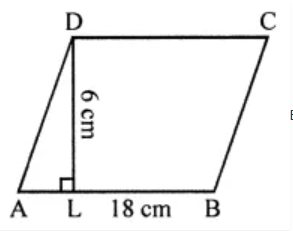# ML Aggarwal Solutions Class 8 Mathematics Solutions for Mensuration Exercise 18.1 in Chapter 18 - Mensuration

A flooring tile has a shape of a parallelogram whose base is 18 cm and the corresponding height is 6 cm. How many such tiles are required to cover a floor of area 540 m2? (If required you can split the tiles in whatever way you want to fill up the comers).

Given,Base of the parallelogram-shaped flooring tile = 18 cm and its height = 6 cm

So,

Area of one tile = Base × Height

= 18 × 6

= 108 cm2

We have the area of floor = 540 m2

Hence, the number of tiles = Total area/ Area of one tile

= (540 x 100 x 100)/108  [[As, 1 m2 = (100 x 100) cm2]]

= 50000

Related Questions

Lido

Courses

Teachers

Book a Demo with us

Syllabus

Maths
CBSE
Maths
ICSE
Science
CBSE

Science
ICSE
English
CBSE
English
ICSE
Coding

Terms & Policies

Selina Question Bank

Maths
Physics
Biology

Allied Question Bank

Chemistry
Connect with us on social media!